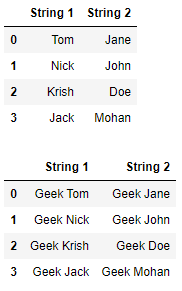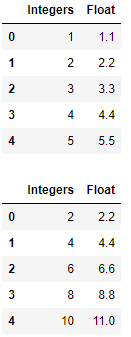Related Articles

# How to Apply a function to multiple columns in Pandas?

• Last Updated : 20 Aug, 2020

Let us see how to apply a function to multiple columns in a Pandas DataFrame. To execute this task will be using the `apply()` function.

## pandas.DataFrame.apply

This function applies a function along an axis of the DataFrame.

Syntax : DataFrame.apply(parameters)

Parameters :

• func : Function to apply to each column or row.
• axis : Axis along which the function is applied
• raw : Determines if row or column is passed as a Series or ndarray object.
• result_type : ‘expand’, ‘reduce’, ‘broadcast’, None; default None
• args : Positional arguments to pass to func in addition to the array/series.
• **kwds : Additional keyword arguments to pass as keywords arguments to func.

Returns : Series or DataFrame

Example 1 : Prepending “Geek” before every element in two columns.

 `# imnport the module``import` `pandas as pd`` ` `# creating a DataFrame``df ``=` `pd.DataFrame({``'String 1'` `:[``'Tom'``, ``'Nick'``, ``'Krish'``, ``'Jack'``], ``                   ``'String 2'` `:[``'Jane'``, ``'John'``, ``'Doe'``, ``'Mohan'``]})`` ` `# displaying the DataFrame``display(df)`` ` `# function for prepending 'Geek'``def` `prepend_geek(name):``    ``return` `'Geek '` `+` `name`` ` `# executing the function``df[[``"String 1"``, ``"String 2"``]] ``=` `df[[``"String 1"``, ``"String 2"``]].``apply``(prepend_geek)`` ` `# displaying the DataFrame``display(df)`

Output :Example 2 : Multiplying the value of each element by 2

 `# imnport the module``import` `pandas as pd`` ` `# creating a DataFrame``df ``=` `pd.DataFrame({``'Integers'` `:[``1``, ``2``, ``3``, ``4``, ``5``], ``                   ``'Float'` `:[``1.1``, ``2.2``, ``3.3``, ``4.4` `,``5.5``]})`` ` `# displaying the DataFrame``display(df)`` ` `# function for prepending 'Geek'``def` `multiply_by_2(number):``    ``return` `2` `*` `number`` ` `# executing the function``df[[``"Integers"``, ``"Float"``]] ``=` `df[[``"Integers"``, ``"Float"``]].``apply``(multiply_by_2)`` ` `# displaying the DataFrame``display(df)`

Output :Attention geek! Strengthen your foundations with the Python Programming Foundation Course and learn the basics.

To begin with, your interview preparations Enhance your Data Structures concepts with the Python DS Course. And to begin with your Machine Learning Journey, join the Machine Learning – Basic Level Course

My Personal Notes arrow_drop_up